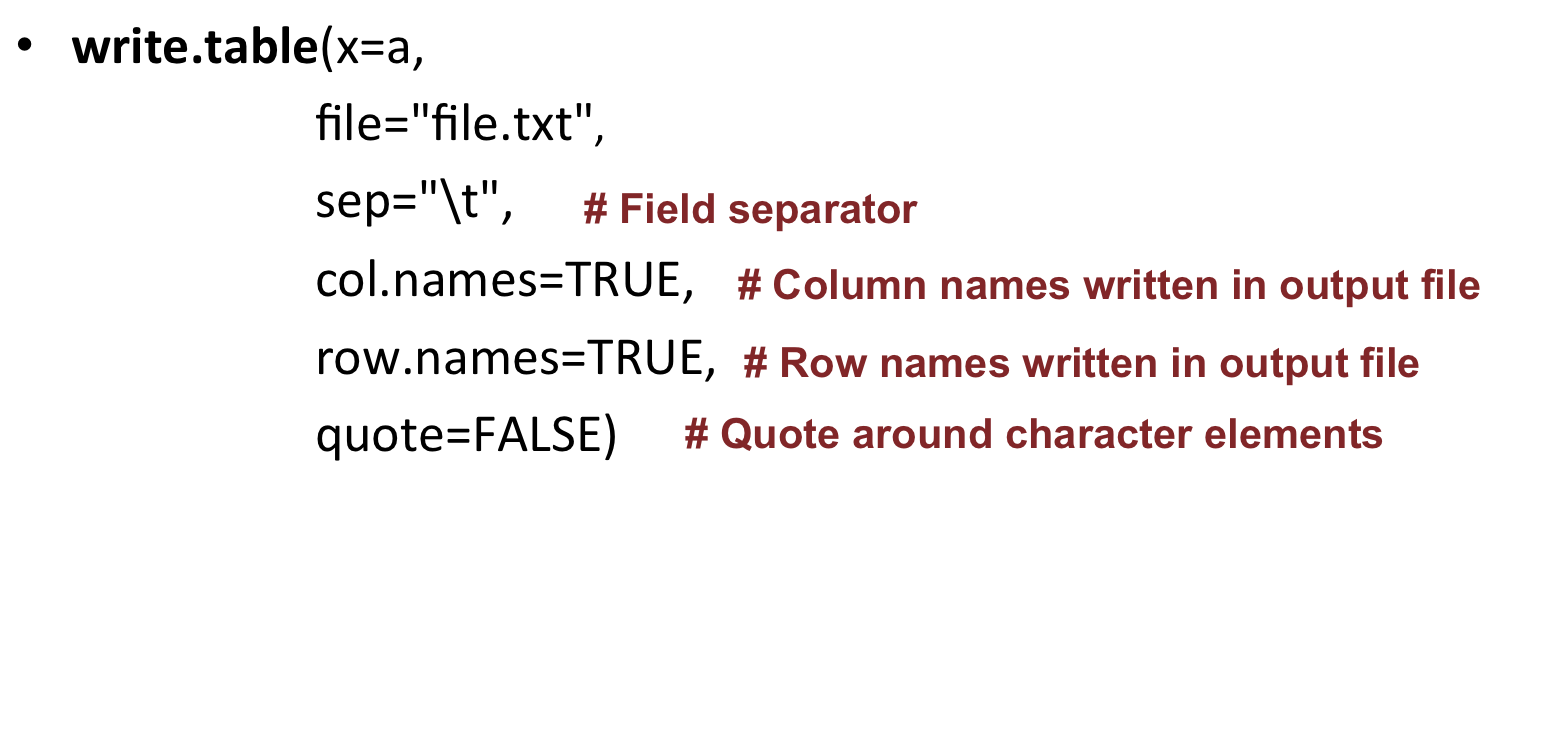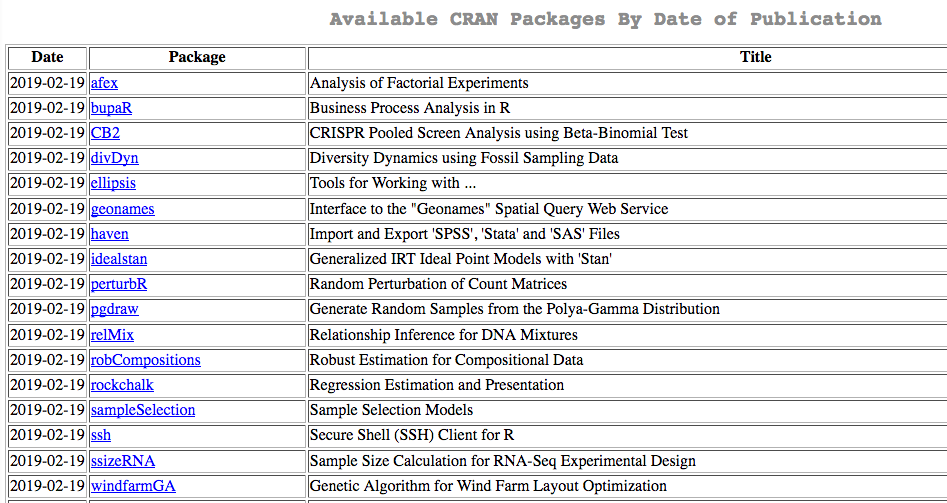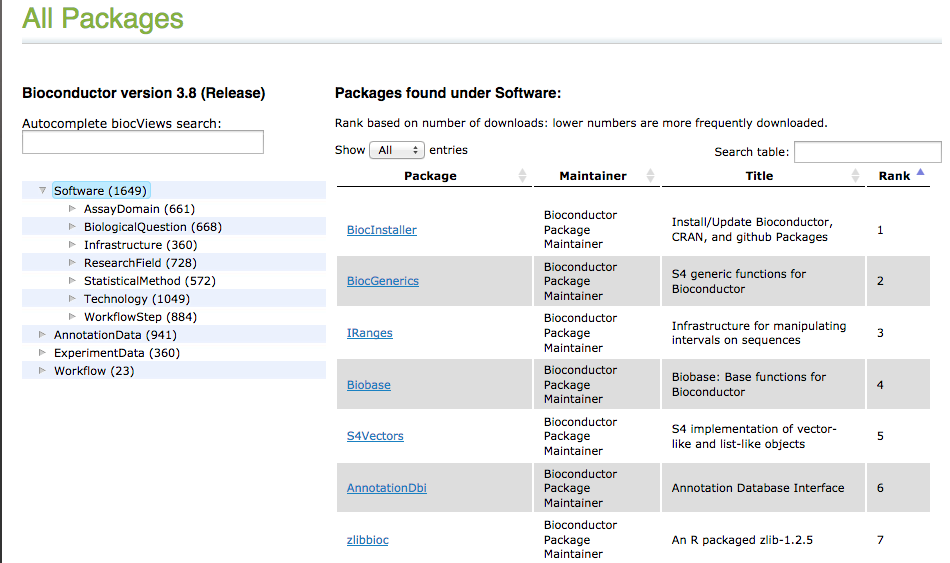# Reviewing some R basics

Open the RStudio software.

## Basics

### Objects

Everything that stores any kind of data in R is an object.

• Assignment operators
• <- or =
• Essentially the same but, to avoid confusions: + Use <- for assignments
• Keep = for functions arguments
• Assigning a value to the object B:
``````B <- 10
``````
• Reassigning: modifying the content of an object:
``````B + 10
``````

B unchanged !!

``````B <- B + 10
``````

B changed !!

• You can see the objects you created in the upper right panel in RStudio: the environment.

### Data types and data structures

Each object has a data type:

• Numeric (number - integer or double)
• Character (text)
• Logical (TRUE / FALSE)

Number:

``````a <- 10
mode(a)
typeof(a)
str(a)
``````

Text:

``````b <- "word"
mode(b)
typeof(b)
str(b)
``````

The main data structures in R are:

• Vector
• Factor
• Matrix
• Data frame

Create a vector:

``````a <- 1:5
``````

Create a second vector, and check with elements of that second vector are also present in a with %in%:

``````b <- 3:8

b[b %in% a]
``````

Check the length of (=number of elements in) a vector:

``````length(b)
``````

Create a data frame:

``````# stringsAsFactors: ensures that characters are treated as characters and not as factors
d <- data.frame(Name=c("Maria", "Juan", "Alba"),
Age=c(23, 25, 31),
Vegetarian=c(TRUE, TRUE, FALSE),
stringsAsFactors = FALSE)
``````

Check dimensions of a dataframe:

``````# Number of rows
nrow(d)

# Number of columns
ncol(d)

# Dimensions (first element is the number of rows, second element is the number of columns)
dim(d)
``````

Select rows of the data frame if the Age column is superior to 24:

``````d[d\$Age > 24,]
``````

Select rows of the data frame if the Age column is superior to 24 AND if Vegetarian is TRUE :

``````d[d\$Age > 24 & d\$Vegetarian == TRUE,]
``````

## Paths and directories

• Get the path of the current directory (know where you are working at the moment) with getwd (get working directory):
``````getwd()
``````
• Change working directory with setwd (set working directory)
Go to a directory giving the absolute path:
``````setwd("~/rnaseq_course")
``````

Go to a directory giving the relative path:

``````setwd("differential_expression")
``````

You are now in: “~/rnaseq_course/differential_expression”
Move one directory “up” the tree:

``````setwd("..")
``````

You are now in: “~/rnaseq_course”

## Missing values

NA (Not Available) is a recognized element in R.

• Finding missing values in a vector
``````# Create vector
x <- c(4, 2, 7, NA)

# Find missing values in vector:
is.na(x)

# Remove missing values
na.omit(x)
x[ !is.na(x) ]
``````
• Some functions can deal with NAs, either by default, or with specific arguments:
``````x <- c(4, 2, 7, NA)

# default arguments
mean(x)

# set na.rm=TRUE
mean(x, na.rm=TRUE)
``````
• In a matrix or a data frame, keep only rows where there are no NA values:
``````# Create matrix with some NA values
mydata <- matrix(c(1:10, NA, 12:2, NA, 15:20, NA), ncol=3)

# Keep only rows without NAs
mydata[complete.cases(mydata), ]
# or
na.omit(mydata)
``````

Check this R blogger post on missing/null values

### On vectors

• Read a file as a vector with the scan function
``````# Read in file
scan(file="file.txt")
# Save in  object
k <- scan(file="file.txt")
``````

By default, scans “double” (numeric) elements: it fails if the input contains characters.
If non-numeric, you need to specify the type of data contained in the file:

``````# specify the type of data to scan
scan(file="file.txt",
what="character")
scan(file="~/file.txt",
what="character")
``````

Regarding paths of files:
If the file is not in the current directory, you can provide a full or relative path. For example, if located in the home directory, read it as:

``````scan(file="~/file.txt",
what="character")
``````
• Write the content of a vector in a file:
``````# create a vector
mygenes <- c("SMAD4", "DKK1", "ASXL3", "ERG", "CKLF", "TIAM1", "VHL", "BTD", "EMP1", "MALL", "PAX3")
# write in a file
write(x=mygenes,
file="gene_list.txt")
``````

Regarding paths of files:
When you write a file, you can also specify a full or relative path:

``````# Write to home directory
write(x=mygenes,
file="~/gene_list.txt")
# Write to one directory up
write(x=mygenes,
file="../gene_list.txt")
``````

### On data frames or matrices

• Read in a file into a data frame with the read.table function:
``````a <- read.table(file="file.txt")
``````

You can convert it as a matrix, if needed, with:

``````a <- as.matrix(read.table(file="file.txt"))
``````
• Write a data frame or matrix to a file:
``````write.table(x=a,
file="file.txt")
``````

Useful arguments:• Note that “\t” stands for tab-delimitation

## Install packages

### R base

A set a standard packages which are supplied with R by default.
Example: package base (write, table, rownames functions), package utils (read.table, str functions), package stats (var, na.omit, median functions).

### R contrib

All other packages:

• CRAN: Comprehensive R Archive Network + 15356* packages available + find packages in https://cran.r-project.org/web/packages/• Bioconductor: + 1823* packages available + find packages in https://bioconductor.org/packages*As of February 2020

Install a CRAN package using install.packages:

``````install.packages('BiocManager', repos = 'http://cran.us.r-project.org', dependencies = TRUE)
``````

Install a Bioconductor package using BiocManager::install:

``````library('BiocManager')
BiocManager::install('GOstats')
``````

## Exercise: warming up !

• Ex1.
• Create a numeric vector y which contains the numbers from 2 to 11, both included.
• How many elements are in y? I.e what is the length of vector y ?
• Show the 3rd and the 6th elements of y.
• Show all elements of y that have a value inferior to 7.
• Ex2.
• Create the vector x of 1000 random numbers from the normal distribution (with rnorm).
• What are the mean, median, minimum and maximum values of x?
• Ex3.
• Create vector y2 as: y2 <- c(1, 11, 5, 62, NA, 18, 2, 8, NA)
• What is the sum of all elements in y2 ?
• Which elements of y2 are also present in y?
• Remove NA values from y2.
• Ex4.
• Create the following data frame:
 43 181 M 34 172 F 22 189 M 27 167 F

with row names: John, Jessica, Steve, Rachel and column names: Age, Height, Sex.

• Check the structure of df with str().
• Calculate the average age and height in df.
• Change the row names of df so the data becomes anonymous (use for example Patient1, Patient2, etc.)
• Write df to the file mydf.txt with write.table(). Explore parameters sep, row.names, col.names, quote.

CORRECTION

``````
# Ex1.
#Create a numeric vector y which contains the numbers from 2 to 11, both included.
y <- 2:11
# same as y <- c(2, 3, 4, 5, 6, 7, 8, 9, 10, 11)
#How many elements are in y? I.e what is the length of vector y ?
length(y)
#Show the 3rd and the 6th elements of y.
y[c(3, 6)]
#Show all elements of y that have a value inferior to 7.
y[y < 7]

# Ex2.
#Create the vector x of 1000 random numbers from the normal distribution (with rnorm).
x <- rnorm(1000)
#What are the mean, median, minimum and maximum values of x?
mean(x); median(x); min(x); max(x)
# more straightforward:
summary(x)

# Ex3.
#Create vector y2 as: y2 <- c(1, 11, 5, 62, NA, 18, 2, 8, NA)
y2 <- c(1, 11, 5, 62, NA, 18, 2, 8, NA)
#What is the sum of all elements in y2 ?
sum(y2, na.rm = TRUE)
# same as sum(na.omit(y2))
#Which elements of y2 are also present in y?
y2[y2 %in% y]
#Remove NA values from y2.
y2 <- na.omit(y2)

# Ex4.
#Create the following data frame (I will call it df):
#with row names: John, Jessica, Steve, Rachel
#and column names: Age, Height, Sex.
df <- data.frame(Age=c(43, 34, 22, 27),
Height=c(181, 172, 189, 167),
Sex=c("M", "F", "M", "F"),
row.names = c("John", "Jessica", "Steve", "Rachel"))
#Check the structure of df with str().
str(df)
#Calculate the average age and height in df.
mean(df\$Age) # same as mean(df[,"Age"])
mean(df\$Height) # same as mean(df[,"Height"])
# or
colMeans(df[,c("Age", "Height")])
#Change the row names of df so the data becomes anonymous
# (use for example Patient1, Patient2, etc.)
rownames(df) <- c("Patient1", "Patient2", "Patient3", "Patient4")
#Write df to the file mydf.txt with write.table().
# Explore parameters sep, row.names, col.names, quote.
write.table(df,
"mydf.txt",
sep="\t",
row.names = TRUE,
col.names = NA,
quote = FALSE)
``````